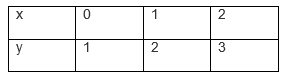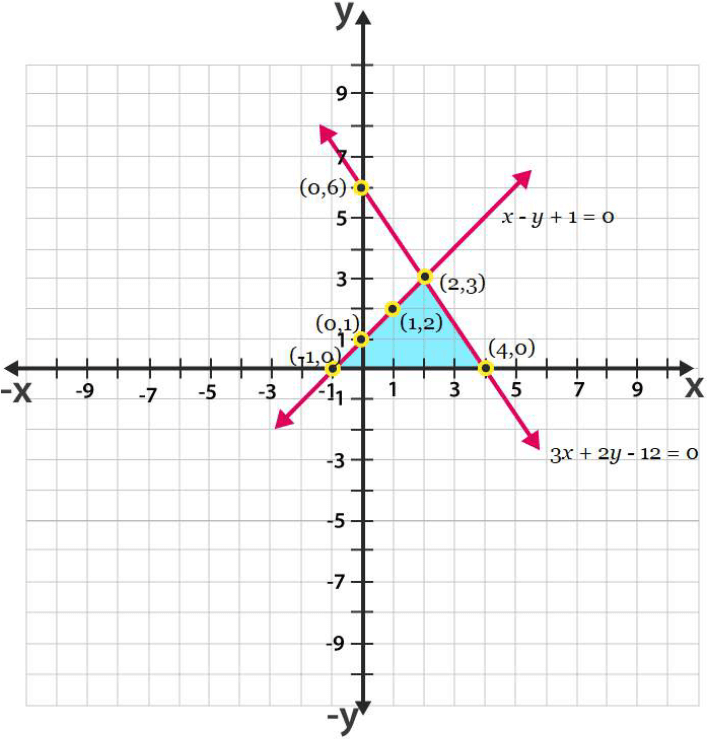Guru

# Draw the graphs of the equations x – y + 1 = 0 and 3x + 2y – 12 = 0. Determine the coordinates of the vertices of the triangle formed by these lines and the x-axis, and shade the triangular region. Q.7

• 0

What is the solution of the question of class 10th math of exercise no. 3.2. of question no.7. By how many ways we can solve this question. Please help me for solving this question Draw the graphs of the equations x – y + 1 = 0 and 3x + 2y – 12 = 0. Determine the coordinates of the vertices of the triangle formed by these lines and the x-axis, and shade the triangular region.

Share

1. Given, the equations for graphs are x – y + 1 = 0 and 3x + 2y – 12 = 0.

For, x – y + 1 = 0 or x = 1+yFor, 3x + 2y – 12 = 0 or x = (12-2y)/3Hence, the graphical representation of these equations is as follows;From the figure, it can be seen that these lines are intersecting each other at point (2, 3) and x-axis at (−1, 0) and (4, 0). Therefore, the vertices of the triangle are (2, 3), (−1, 0), and (4, 0).

• 0